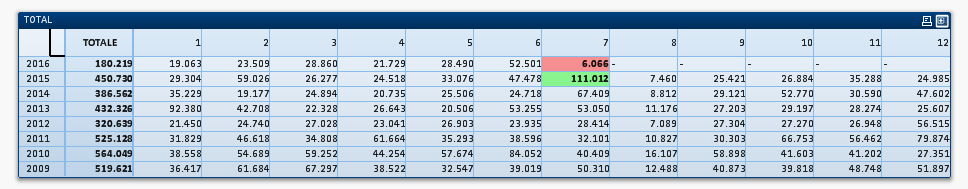Announcements
MAINTENANCE ALERT: Dec. 12th starting 9 AM CET. Community will be read-only. GET DETAILS
cancel
Showing results for
Did you mean:Creator

## Highlight max and min values in pivot table

Hi, I need help to correct highlight max and min values in pivot table

In attached document i use in background color setting

if(isnull(sum(W_TOT_KG)) <> -1,
if(sum(W_TOT_KG) = Max(total aggr(sum(W_TOT_KG), MESE_DOC, ANNO_DOC)), rgb(0,255,0),
If(IsNull(sum(W_TOT_KG) ) <> -1 and sum(W_TOT_KG) <> 0,
If(sum(W_TOT_KG) = Min(total aggr(
If(ISNULL(sum(W_TOT_KG)) <> -1 AND sum(W_TOT_KG) <> 0,
sum(W_TOT_KG)
)
,MESE_DOC, ANNO_DOC)), rgb(255,0,0))
)
)
)

but it does not always work

Can someone help me?

Thank you very much

1 Solution

Accepted SolutionsMaster III

Try

If(Sum(W_TOT_KG)=Min({<MESE_DOC={"=Sum(W_TOT_KG) > 0"}>} TOTAL Aggr(Sum(W_TOT_KG),ANNO_DOC,MESE_DOC)),Red(),If(Sum(W_TOT_KG)=Max(TOTAL Aggr(Sum(W_TOT_KG),ANNO_DOC,MESE_DOC)),Green()))

19 RepliesMVP

Seems to be working, no? What is the issue here?Creator
Author

in this case it works, but sometimes it does not highlight the values correctly.Master III

Hi Paolo,

Your problem is MESE_Doc = 0.

Change Dimension

If(MESE_Doc > 0,MESE_DOC)

and Check 'Suppress when value is Null'

In Background Color Setting :

If(RangeMin(Top(Sum(W_TOT_KG),1,NoOfRows()))=Sum(W_TOT_KG),Red(),If(RangeMax(Top(Sum(W_TOT_KG),1,NoOfRows()))=Sum(W_TOT_KG),Green()))

See Attachment

Regards,

AntonioMVP

What selections have you made here? I am unable to replicate the problem at my endCreator
Author

Thank you, your solution highlight the maximum and minimum values for each column.

I need to highlight only the cell with the maximum value of the entire pivot and

the cell with the minimum value of the entire pivot

Thank you very muchMaster III

Try

If(Sum(W_TOT_KG)=Min({<W_TOT_KG-={0}>} TOTAL Aggr(Sum(W_TOT_KG),ANNO_DOC,MESE_DOC)),Red(),If(Sum(W_TOT_KG)=Max(TOTAL Aggr(Sum(W_TOT_KG),ANNO_DOC,MESE_DOC)),Green()))Creator
Author

The problem occurs randomly and do not understand when. I think there is something wrong
in the formula I use in Background Color Setting when I have so many records.

The problem occurs on a very heavy QlikView document and I find
it hard to reproduce in a test version

Thank you very muchMVP

Can you try this may beCreator
Author

Sign - between Min({<W_TOT_KG and ={0}>} is correct?

I remove it and I tried your new solution, but not always workCommunity Browser